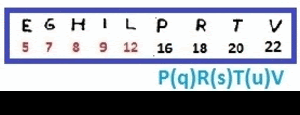## HERVPILTG : LEIGH PRTV## sexta-feira, 5 de maio de 2017

### Z 13 SYMMETRY and Binary code theory

Hello,
Another analysis of z13 (my name is), this time with no result, I just observed a pattern (that must have already been seen by others), but I share, because who knows someone can not see anything else.
That's the pattern.
There are Alphabetical symbols and non-alphabetic symbols,
Note that they are distributed symmetrically.

Being an alternate coding (if that's really something)
Example, a binary code (A = Alpha, S = Symbol)
If A = 1 and S = 0

We would have

1110010100111

binary converter here

Which in decimal is

7335

If A = 0 and S = 1

We would have

0001101011000

Which in decimal is

856

These numbers mean nothing (as far as I know), is just an example
The most curious, in fact, to me is the symmetry of the distribution of the symbols.

Marcelo